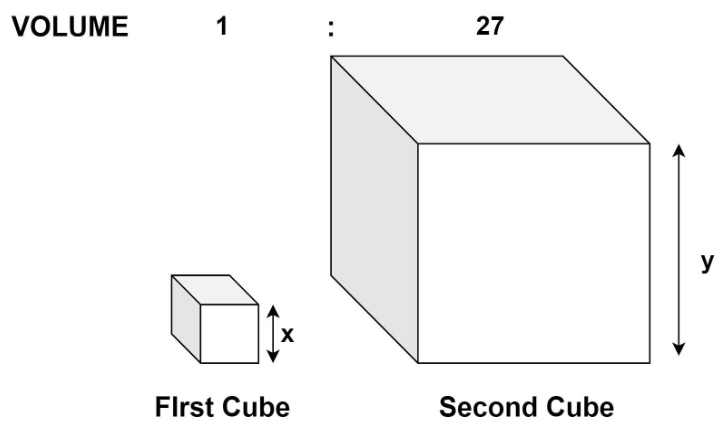QuestionAnswers

# Two cubes have their volumes in the ratio 1:27. Find the ratio of their surface areas.A) 3:9B) 1:8C) 1:9D) 2:9Hint: The ratio of the volume of the cubes can be used to calculate the ratio of the sides. After obtaining the ratio of sides, the ratio of surface area can be easily determined.

Given in the problem, two cubes have their volumes in the ratio 1:27.
We need to find the ratio of their surface areas.
In geometry, a cube is a three-dimensional solid object bounded by six square faces, facets or sides, with three meeting at each vertex.
If a cube has a side $x {\text { unit}}$, then the volume of the cube is given by ${x^3}{\left( \text {unit} \right)^3}$.
Also, the surface area of the cube is given by $6{x^2}{\left( \text {unit} \right)^2}$.
In the problem there are two cubes given.
Let the side of the first cube be $x{\text{ }}unit$ and that of the second be $y{\text{ }}unit$ respectively.Hence, the volume of the first cube will be ${x^3}{\left( \text{unit} \right)^3}$.
And the volume of the second cube will be ${y^3}{\left( \text {unit} \right)^3}$.
It is given that the ratio of their volumes is 1:27.
$\Rightarrow \dfrac{{{\text{Volume of first cube}}}}{{{\text{Volume of second cube}}}} = \dfrac{1}{{27}} \\ \Rightarrow \dfrac{{{x^3}}}{{{y^3}}} = {\left( {\dfrac{x}{y}} \right)^3} = \dfrac{1}{{27}} \\ \Rightarrow \dfrac{x}{y} = {\left( {\dfrac{1}{{27}}} \right)^{\dfrac{1}{3}}} = \dfrac{1}{3} ………....(1) \\$
Hence the ratio of the side of the first cube to that of the second cube is 1:3.
We need to find the ratio of their surface area.
By using the above-mentioned formula, we get,
Surface area of the first cube $= 6{x^2}{\left( \text {unit} \right)^2}$
Surface area of the second cube $= 6{y^2}{\left( \text {unit} \right)^2}$
Therefore, ratio of their surface area is given by
$\Rightarrow \dfrac{{{\text{Surface area of first cube}}}}{{{\text{Surface area of second cube}}}} = \dfrac{{6{x^2}}}{{6{y^2}}} = {\left( {\dfrac{x}{y}} \right)^2}$
Using equation (1) in the above, we get
$\Rightarrow \dfrac{{{\text{Surface area of first cube}}}}{{{\text{Surface area of second cube}}}} = {\left( {\dfrac{x}{y}} \right)^2} = {\left( {\dfrac{1}{3}} \right)^2} = \dfrac{1}{9}$
Hence the ratio of surface area of the first cube to that of the second cube is 1:9.
Therefore, option (C). 1:9 is the correct answer.

Note: The formula of volume and surface area of the cube should be kept in mind while solving problems like above. Ratio is the quantitative relation between two amounts showing the number of times one value contains or is contained within the other. The unit of both the quantities in the ratio should be the same. In problems like the above effort should be made to obtain the desired result while assuming the minimum number of unknown quantities.
CBSE Class 10 Maths Chapter 13 - Surface Areas and Volumes FormulaCBSE Class 8 Maths Chapter 7 - Cubes and Cube Roots FormulasWhy do we Have Two Eyes?CBSE Class 10 Maths Chapter 3 - Pair of Linear Equations in Two Variables FormulaCBSE Class 9 Maths Chapter 13 - Surface Areas and Volumes FormulasRespiratory and Lung VolumesCBSE Class 6 Maths Chapter 12 - Ratio and Proportion FormulasPhysical Properties of Alkanes and Their VariationsPair of Linear Equation in Two VariablesExcretory Products and Their EliminationImportant Questions for CBSE Class 10 Maths Chapter 3 - Pair of Linear Equations in Two VariablesImportant Questions for CBSE Class 8 Maths Chapter 7 - Cubes and Cube RootsCBSE Class 6 Science The Living Organisms and Their Surroundings WorksheetsImportant Questions for CBSE Class 6 English A Pact with The Sun Chapter 1 - A Tale of Two BirdsImportant Questions for CBSE Class 10 Maths Chapter 13 - Surface Areas and VolumesImportant Questions for CBSE Class 9 Maths Chapter 4 - Linear Equations in Two VariablesImportant Questions for CBSE Class 6 Science Chapter 9 - The Living Organisms and Their SurroundingsImportant Questions for CBSE Class 6 Maths Chapter 12 - Ratio and ProportionImportant Questions for CBSE Class 9 Maths Chapter 13 - Surface Areas and VolumesImportant Questions for CBSE Class 6 English A Pact with The Sun Chapter 10 - A Strange Wrestling MatchCBSE Class 10 Maths Question Paper 2017CBSE Class 10 Hindi A Question Paper 2020Hindi A Class 10 CBSE Question Paper 2009Hindi A Class 10 CBSE Question Paper 2015CBSE Class 10 Maths Question Paper 2020Hindi A Class 10 CBSE Question Paper 2016Hindi A Class 10 CBSE Question Paper 2012Hindi A Class 10 CBSE Question Paper 2010Hindi A Class 10 CBSE Question Paper 2007Hindi A Class 10 CBSE Question Paper 2013RD Sharma Solutions for Class 10 Maths Chapter 16 - Surface Areas and VolumesNCERT Solutions for Class 10 Maths Chapter 13 Surface Areas and VolumesNCERT Solutions for Class 8 Maths Chapter 7 Cubes and Cube RootsNCERT Solutions for Class 9 Maths Chapter 13 Surface Areas and VolumesNCERT Solutions for Class 8 Maths Chapter 7 Cubes and Cube Roots in HindiNCERT Solutions for Class 10 Maths Chapter 3 Pair of Linear Equations in Two Variables in HindiNCERT Solutions for Class 10 Maths Chapter 3 Pair of Linear Equations in Two VariablesNCERT Solutions for Class 10 Maths Chapter 13 Surface Areas and Volumes in HindiRD Sharma Solutions for Class 10 Maths Chapter 3 - Pair of Linear Equations in Two VariablesRS Aggarwal Class 10 Solutions - Linear Equations in two variables